Updating search results...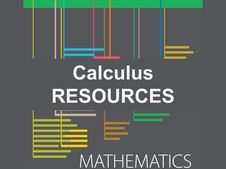# Calculus Resources

This collection contains highly recommended Calculus lessons, activities, and other resources from the eMedia library.

19 affiliated resources

# Search Resources

View
Selected filters: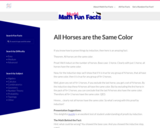Restricted Use
Rating
0.0 stars

This Math Fun Fact focuses on proof by induction.
Math Fun Facts were developed as warm-up activities. They are mathematical tidbits meant to arouse curiosity and fascination with the subject. Fun Facts give students a glimpse that mathematics is full of interesting ideas, patterns, and new modes of thinking.

Subject:
Mathematics
Material Type:
Activity/Lab
Provider:
Mudd Math Fun Facts
Author:
Francis Su
12/01/2022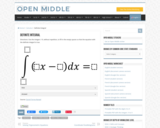Restricted Use
Rating
0.0 stars

Open Middle provides math problems that have a closed beginning, a closed end, and an open middle. This means that there are multiple ways to approach and ultimately solve the problems. Open middle problems generally require a higher Depth of Knowledge than most problems that assess procedural and conceptual understanding.

Subject:
Mathematics
Material Type:
Activity/Lab
Provider:
Open Middle Math
04/26/2022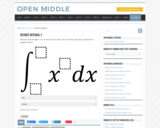Restricted Use
Rating
0.0 stars

Open Middle provides math problems that have a closed beginning, a closed end, and an open middle. This means that there are multiple ways to approach and ultimately solve the problems. Open middle problems generally require a higher Depth of Knowledge than most problems that assess procedural and conceptual understanding.

Subject:
Mathematics
Material Type:
Activity/Lab
Provider:
Open Middle Math
04/26/2022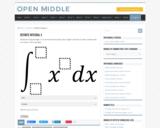Restricted Use
Rating
0.0 stars

Open Middle provides math problems that have a closed beginning, a closed end, and an open middle. This means that there are multiple ways to approach and ultimately solve the problems. Open middle problems generally require a higher Depth of Knowledge than most problems that assess procedural and conceptual understanding.

Subject:
Mathematics
Material Type:
Activity/Lab
Provider:
Open Middle Math
04/26/2022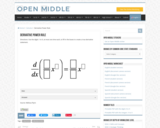Restricted Use
Rating
0.0 stars

Open Middle provides math problems that have a closed beginning, a closed end, and an open middle. This means that there are multiple ways to approach and ultimately solve the problems. Open middle problems generally require a higher Depth of Knowledge than most problems that assess procedural and conceptual understanding.

Subject:
Mathematics
Material Type:
Activity/Lab
Provider:
Open Middle Math
04/26/2022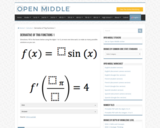Restricted Use
Rating
0.0 stars

Open Middle provides math problems that have a closed beginning, a closed end, and an open middle. This means that there are multiple ways to approach and ultimately solve the problems. Open middle problems generally require a higher Depth of Knowledge than most problems that assess procedural and conceptual understanding.

Subject:
Mathematics
Material Type:
Activity/Lab
Provider:
Open Middle Math
04/26/2022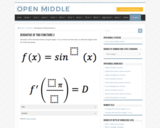Restricted Use
Rating
0.0 stars

Open Middle provides math problems that have a closed beginning, a closed end, and an open middle. This means that there are multiple ways to approach and ultimately solve the problems. Open middle problems generally require a higher Depth of Knowledge than most problems that assess procedural and conceptual understanding.

Subject:
Mathematics
Material Type:
Activity/Lab
Provider:
Open Middle Math
04/26/2022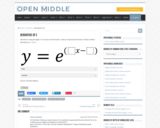Restricted Use
Rating
0.0 stars

Open Middle provides math problems that have a closed beginning, a closed end, and an open middle. This means that there are multiple ways to approach and ultimately solve the problems. Open middle problems generally require a higher Depth of Knowledge than most problems that assess procedural and conceptual understanding.

Subject:
Mathematics
Material Type:
Activity/Lab
Provider:
Open Middle Math
04/26/2022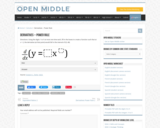Restricted Use
Rating
0.0 stars

Open Middle provides math problems that have a closed beginning, a closed end, and an open middle. This means that there are multiple ways to approach and ultimately solve the problems. Open middle problems generally require a higher Depth of Knowledge than most problems that assess procedural and conceptual understanding.

Subject:
Mathematics
Material Type:
Activity/Lab
Provider:
Open Middle Math
04/26/2022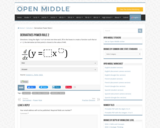Restricted Use
Rating
0.0 stars

Open Middle provides math problems that have a closed beginning, a closed end, and an open middle. This means that there are multiple ways to approach and ultimately solve the problems. Open middle problems generally require a higher Depth of Knowledge than most problems that assess procedural and conceptual understanding.

Subject:
Mathematics
Material Type:
Activity/Lab
Provider:
Open Middle Math
04/26/2022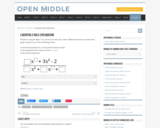Restricted Use
Rating
0.0 stars

Open Middle provides math problems that have a closed beginning, a closed end, and an open middle. This means that there are multiple ways to approach and ultimately solve the problems. Open middle problems generally require a higher Depth of Knowledge than most problems that assess procedural and conceptual understanding.

Subject:
Mathematics
Material Type:
Activity/Lab
Provider:
Open Middle Math
04/26/2022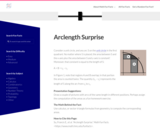Restricted Use
Rating
0.0 stars

This Math Fun Fact focuses on using calculus, or sector-triangle formulas from geometry, to compute corresponding areas.
Math Fun Facts were developed as warm-up activities. They are mathematical tidbits meant to arouse curiosity and fascination with the subject. Fun Facts give students a glimpse that mathematics is full of interesting ideas, patterns, and new modes of thinking.

Subject:
Mathematics
Material Type:
Activity/Lab
Provider:
Mudd Math Fun Facts
Author:
Francis Su
12/01/2022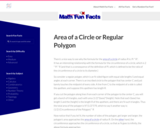Restricted Use
Rating
0.0 stars

This Math Fun Fact focuses on approximating the area of the circle by slicing it into thin wedges, which is analogous to the process of integration (in calculus) to find an area.
Math Fun Facts were developed as warm-up activities. They are mathematical tidbits meant to arouse curiosity and fascination with the subject. Fun Facts give students a glimpse that mathematics is full of interesting ideas, patterns, and new modes of thinking.

Subject:
Mathematics
Material Type:
Activity/Lab
Provider:
Mudd Math Fun Facts
Author:
Francis Su
12/01/2022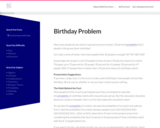Restricted Use
Rating
0.0 stars

This Math Fun Fact focuses on calculating the probability of a match.
Math Fun Facts were developed as warm-up activities. They are mathematical tidbits meant to arouse curiosity and fascination with the subject. Fun Facts give students a glimpse that mathematics is full of interesting ideas, patterns, and new modes of thinking.

Subject:
Mathematics
Material Type:
Activity/Lab
Provider:
Mudd Math Fun Facts
Author:
Francis Su
12/01/2022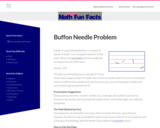Restricted Use
Rating
0.0 stars

This Math Fun Fact focuses on calculating pi and probability theory.
Math Fun Facts were developed as warm-up activities. They are mathematical tidbits meant to arouse curiosity and fascination with the subject. Fun Facts give students a glimpse that mathematics is full of interesting ideas, patterns, and new modes of thinking.

Subject:
Mathematics
Material Type:
Activity/Lab
Provider:
Mudd Math Fun Facts
Author:
Francis Su
12/01/2022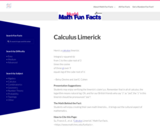Restricted Use
Rating
0.0 stars

This Math Fun Fact focuses on a calculus limerick.
Math Fun Facts were developed as warm-up activities. They are mathematical tidbits meant to arouse curiosity and fascination with the subject. Fun Facts give students a glimpse that mathematics is full of interesting ideas, patterns, and new modes of thinking.

Subject:
Mathematics
Material Type:
Activity/Lab
Provider:
Mudd Math Fun Facts
Author:
Francis Su
12/01/2022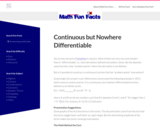Restricted Use
Rating
0.0 stars

This Math Fun Fact focuses on constructing a continuous function.
Math Fun Facts were developed as warm-up activities. They are mathematical tidbits meant to arouse curiosity and fascination with the subject. Fun Facts give students a glimpse that mathematics is full of interesting ideas, patterns, and new modes of thinking.

Subject:
Mathematics
Material Type:
Activity/Lab
Provider:
Mudd Math Fun Facts
Author:
Francis Su
12/01/2022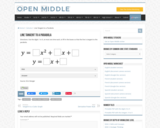Restricted Use
Rating
0.0 stars

Open Middle provides math problems that have a closed beginning, a closed end, and an open middle. This means that there are multiple ways to approach and ultimately solve the problems. Open middle problems generally require a higher Depth of Knowledge than most problems that assess procedural and conceptual understanding.

Subject:
Mathematics
Material Type:
Activity/Lab
Provider:
Open Middle Math
04/26/2022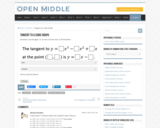Restricted Use